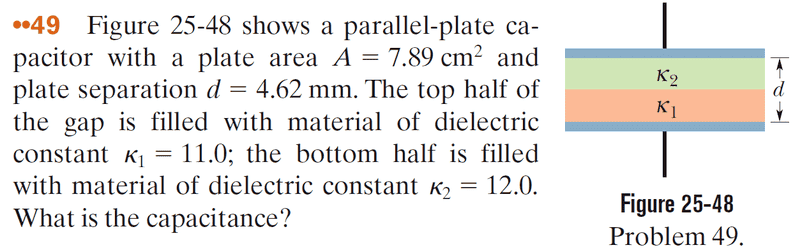# Finding the Potential Difference In Parallel Capacitors

• r0306

#### r0306

Originally posted in a non-homework forum, so the homework template is missing
The question is based on the image below but is not the same. The question is as follows:

Assume the capacitor is charged, so that there is a charge q on the top plate and a charge -q on the bottom plate. Determine the magnitude of the potential difference across the k2 region, answering in units of q. *There is a typo in the book; k1 is the lower half of the gap. Answer in units of 109 V/C (Volt per Coulomb because this factor is multiplied by q, so the Coulomb cancels)My attempt:

I used the parallel capacitor equation E = (Q / A) / 2(epsilon)(k) to find the electric fields for both plates. I added up the two fields and got -5.42*10^11 for the total electric field between the two plates. Then I used the equation E = V / d, plugging in my electric field and using 4.62 * 10^-3 for d and got a change in V of -1.25 * 10^9. I don't know where I went wrong can someone please help me find my mistake? Thanks.

I used the parallel capacitor equation E = (Q / A) / 2(epsilon)(k) to find the electric fields for both plates. I added up the two fields and got -5.42*10^11 for the total electric field between the two plates. Then I used the equation E = V / d, plugging in my electric field and using 4.62 * 10^-3 for d and got a change in V of -1.25 * 10^9. I don't know where I went wrong can someone please help me find my mistake? Thanks.

How did you arrive at a numerical value for the field if you were not given a specific value for q?

A dielectric modifies the net electric field strength inside of it, so the field due to a given plate will be different for the two regions. You'd have to account for that. Further, they're looking for the potential across just one of the dielectric regions and you've used the entire distance between the plates, which would yield a potential difference across the the whole capacitor (if the field were in fact uniform).

An approach that you might consider is to treat the two dielectric regions as individual capacitors, where a 'virtual plate' occupies the boundary between the dielectrics. Then you can calculate the resulting capacitance of each 'capacitor' separately and apply the relationship Q = CV, where Q is the charge on the capacitor, C is the capacitance, and V the potential difference between the plates.

How did you arrive at a numerical value for the field if you were not given a specific value for q?

A dielectric modifies the net electric field strength inside of it, so the field due to a given plate will be different for the two regions. You'd have to account for that. Further, they're looking for the potential across just one of the dielectric regions and you've used the entire distance between the plates, which would yield a potential difference across the the whole capacitor (if the field were in fact uniform).

An approach that you might consider is to treat the two dielectric regions as individual capacitors, where a 'virtual plate' occupies the boundary between the dielectrics. Then you can calculate the resulting capacitance of each 'capacitor' separately and apply the relationship Q = CV, where Q is the charge on the capacitor, C is the capacitance, and V the potential difference between the plates.

The Q values were simply 1 and -1 respectively. Does this mean I should use half the distance given (2.31 mm) then?

EDIT: I tried using half the distance, yielding me a value of -1.25 but the answer is still wrong.

The Q values were simply 1 and -1 respectively. Does this mean I should use half the distance given (2.31 mm) then?
Yes, certainly. You want to use the net field inside the k2 region and determine the potential difference across that region. The k2 region has thickness d/2.
EDIT: I tried using half the distance, yielding me a value of -1.25 but the answer is still wrong.